说起大块头的SUV，大家免不了要说油耗高，但近日，在哈弗F7举办的智能节油挑战赛上，上百位媒体记者用亲身试驾实测证明，并非所有SUV都是“油老虎”，省油的关键不仅靠驾驶技术，更得靠好车！

为了更公正、真实地反映哈弗F7的节油性能，此次节油赛选择了在全国从北至南7大城市进行，通过不同地形地貌、不同气候条件、不同的用车场景进行实地测试。首战北京，哈弗F7就以百公里5.93L的油耗成绩创下纪录，赢得开门红。紧接着石家庄、西安站，在全新的赛制和路况中，哈弗F7分别创下了百公里仅6.45L、5.2L的佳绩。而在成都的“底油”（仅剩约6.8L燃油）测试比拼最长驾驶里程的赛制中，哈弗F7最终交出续航里程长达123公里的成绩单，再度以实测数据验证其出众的节油实力。如此亮眼的赛绩摆在眼前，你是否也想问一把，哈弗F7是怎么做到的？开车如何才能更省油？

汽车的油耗和动力系统关系最为密切，在购车前，如果你希望爱车有着让人羡慕的油耗，笔者认为购车时应该首选配有节油技术的车型。所谓“兵马未动，粮草先行”，哈弗F7通过系列的节油挑战赛告诉大家，选对车才是打好“节油战”的最好证明。

选购技术一：涡轮增压发动机，早已不再新鲜，是既追求动力，又追求低油耗车友的首选。凡是试驾过哈弗F7的朋友都会对其动力表现非常认可，因为哈弗F7搭载了1.5GDIT “中国十佳发动机”和2.0GDIT发动机，2.0GDIT发动机拥有6大先进技术和顶级制造检验标准，应用了许多先进技术，在同级里算是顶尖水准。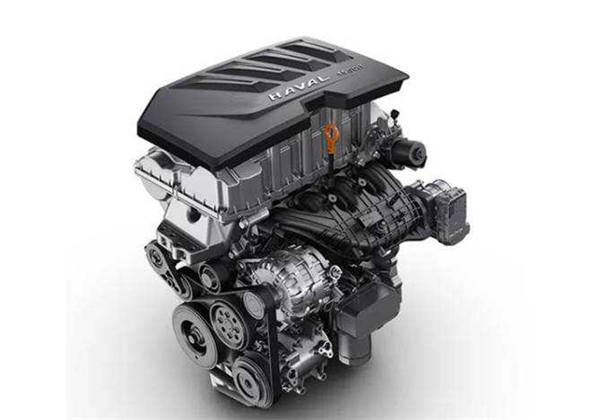选购技术二：全可变气门升程技术，这种技术可以减小发动机泵气损失及机械损失，改善部分负荷的燃油经济性。而哈弗F7的CVVL技术，最高可降低油耗8.4%，同时可提高发动机的动力性、改善加速时的动力瞬态响应性。

选购技术三：哈弗F7的7速湿式双离合变速器，被评为“世界十佳变速器”，与奔驰9AT、大众7DCT相比肩。拥有85项专利和独立知识产权，传达效率高达95%，相比传统7AT变速器，可提升5%的燃油经济性，有效减少油耗，提升换挡效率和平顺性。这款变速器不仅能最大化地减低了动力耗损，助力实现更经济的油耗，并使哈弗F7在频繁起步的工况下依然能保持较高的舒适性。

选购技术四： 发动机智能启停技术，这种技术能在怠速或者等红绿灯时自动关闭发动机，有驾驶需求时自动快速起动发动机的需求，减少了怠速情况下的油耗浪费，提升了燃油经济性。

选购技术五：车身的低风阻设计，能有效降低油耗。哈弗F7流线型车身及悬浮式尾翼设计，风阻系数降低0.043，整车风阻系数0.36，前杠下方气坝设计，能降低燃油0.2L/100km。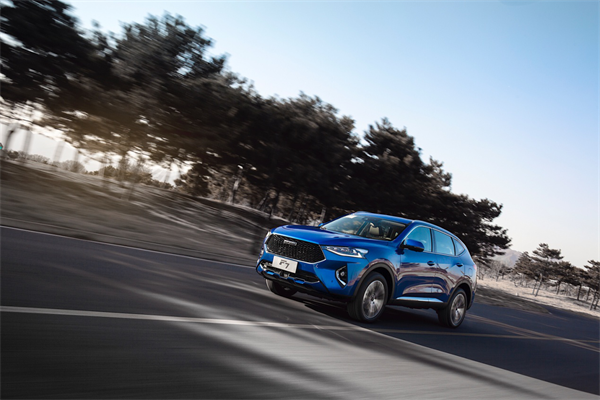作为以自主技术研发为核心的长城汽车，早已注意到汽车性能与节油对于消费者选购的重要性。哈弗F7除了配备上述的节油选购技术，同时还具备多项节油亮点，如中置直喷喷油器，其每个工作循环可以实现多次喷油，不仅保证车子起步时的动力性能，更使燃烧过程更有效，达到节油降耗；二级可变排量机油泵，能实现机油泵高低压模式精准切换，保证发动机具备良好的燃油消耗率；高滚流比进气道与缸盖集成排气歧管双管齐下控制油耗；1.5GDIT发动机采用进、排气双VVT系统，使得缸内燃烧更充分，降低废气污染物的排放，是有颜值有内涵的节油实力派。

笔者一开始也跟很多车主一样，有同样的困惑，大家都是开同样的车，走同样的路，油耗却很不一样。因为每位驾驶者驾驶习惯的差异，造成油耗数据大相径庭。专业的节油教练告诉大家，养成正确的驾驶习惯，开车不仅便利出行，更能为你省下一笔。

一、掌握转速节奏，充分利用每一滴燃油

通常车辆在发动机转数1500-2500左右、时速在60km/h -90km/h时较为省油。但是我们的日常生活中，遇上拥堵路段乃家常便饭，拥堵时时速基本在30-40km/h徘徊，加上频繁的启停，会让油耗迅速飙升。在本次哈弗F7智能节油赛中，北京站首要解决拥堵路况油耗问题。参赛媒体记者凭借自己“老司机”的成熟经验，起步轻踩油门，控制转速在1500转左右，将哈弗F7的黄金动力发挥出最大的节油特性，充分利用每一滴燃油，实现很好的燃油性经济。

在拥堵路段中，除了掌握转速节奏，开启哈弗F7智能启停功能同样能有效抑制油耗的飙升，对节油也很大的帮助。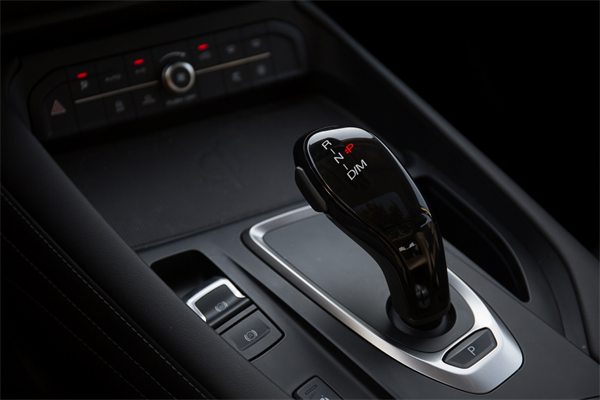二、学会平顺加速，发挥节油最大特性

平日出游，最担心是油灯亮起，而距离附近的加油站还有一段距离，此时还能走多远、汽车抛锚怎么办的问题蜂拥而至。哈弗F7 智能节油赛成都站带领一众媒体记者，亲身经历在油灯已亮的“紧急情况”下（仅剩约6.8L燃油），成功行驶长达123公里，打破了续航焦虑症。成都站的实测告诉我们，日常驾驶碰上油灯亮起时，要记住切勿深踩油门，而是要轻踩油门，尽量用油门的收放来控制车速，保持平顺的加速，则可避免急加速带来的燃油浪费，还能发挥出节油的最大特性。

三、开启ECO节能模式，助力燃油高效运用

当遇到拥堵路况，很多车主都想迅速完成变道超车，所以下意识深踩油门，紧急刹车的情况屡见不鲜，其实这种经常急加速、急减速是驾驶中最费油的坏习惯。其中在南京站比赛中，试驾车队从一开始的镇区路段，就打开了智能启停功能，再配合控制转速、平顺加速的技巧，成功以一个较低油耗通过第一个赛点。但是行至高速路段，没有了红绿灯、拥堵也减少了，智能启停功能在这起不到任何作用。而此时，ECO经济模式和ACC自适应巡航功能便能发挥最大效益。开启哈弗F7的ACC自适应巡航功能，车辆会根据车距自行调节车速向前行驶，只需要双手握住方向盘即可，脚可以从刹车和油门上解放出来，其中哈弗F7配备的12项主动安全科技中FCW前碰撞预警系统和AEB自动紧急制动系统，能提前预判避免频繁刹车和急加速浪费燃油，把油耗控制到最低，保证燃油高效运用。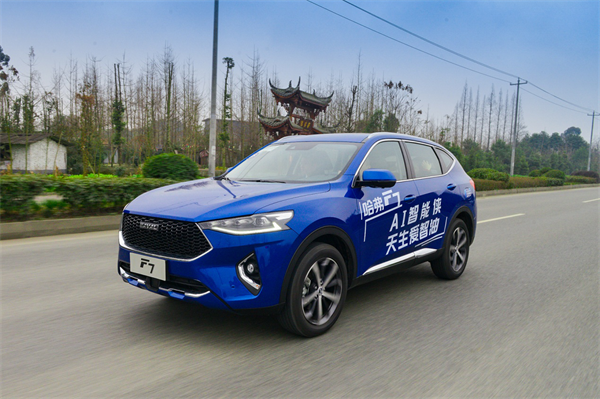四、降低风阻，实现油耗最小化

当汽车在高速上行驶至80km/h时，它有60%-70%的油耗是用来克服风阻。行驶至在高速路上时，原本低风阻设计的车身再关上车窗，不仅能降低胎噪，提升驾驶的静谧性，更有效减少风阻带来的油耗，发挥汽车高速行驶的最大节油特性。

通过哈弗F7智能节油挑战赛的活动，笔者确实感受到不可思议，毕竟对于一台长4620mm、宽1846mm、高1690mm、轴距达2725mm堪称庞然大物的SUV来说，6升以下的油耗压根是不敢想象的，这也证明了哈弗F7在节油技术和产品实力方面的深厚功力可见一斑。更让笔者惊喜的是，作为哈弗F系最新力作，哈弗F7上市仅两个月，销量便达到17109台，成为哈弗汽车突破500万销量的生力军。哈弗F7以优异的销量和惊人的低油耗成绩，用实践证明了自己的产品硬实力。与此同时，在1月13日，哈弗全球500万盛典暨战略发布会在保定哈弗技术中心隆重召开，来自全球哈弗车主、中外专家、合作伙伴及超500家媒体齐聚一堂，共同见证哈弗汽车成为中国首个迈进500万俱乐部的专业SUV品牌，展现出哈弗向全球化进程迈出里程碑意义的一步。500万的新起点，哈弗F7的成功，让我们看到了哈弗汽车领跑中国、逐鹿全球的雄厚实力和战略蓝图，哈弗“5-2-1”战略的实现指日可待。

最后，笔者还想提醒各位车友们，除了上述的选车秘诀和节油驾驶技术，我们在出行之前应该规划好路线，避开拥堵，能为我们的爱车更省点油，让我们的出行更尽“擎”。

##### 相关资讯

|分享

•已阅！握爪
•我手滑为你点赞
•128个赞！
•不明觉厉
•阅后既醉
•有钱！任性
•照片太美，我不敢看
•干货！杠杠的
•高大上
•膜拜中！
•请接受我的膝盖
•猫哥/猫妹么么哒
•神吐槽
•我只笑笑不说话
•我想静静
•窒息
##### 资讯相关车系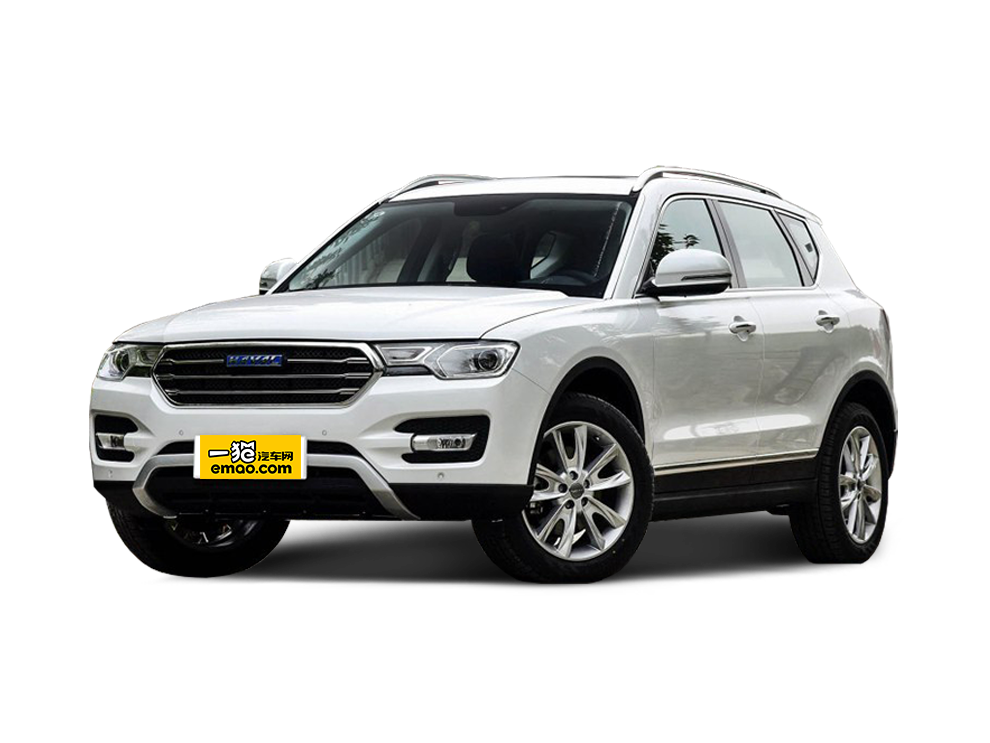##### 热度排行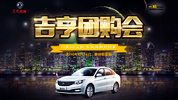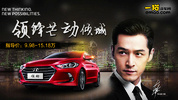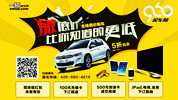• 阿斯顿·马丁
• 奥迪
• 阿尔法罗密欧
• ALPINA
• 爱驰
• AC Schnitzer

• 巴博斯
• 宝骏
• 宝马
• 保时捷
• 北汽制造
• 奔驰
• 奔腾
• 本田
• 比亚迪
• 标致
• 别克
• 宾利
• 布加迪
• 北汽威旺
• 北京
• 北汽绅宝
• 北汽幻速
• 北汽新能源
• 宝沃
• 比速汽车
• 北汽道达
• 北京汽车
• 比德文汽车
• 铂驰
• 北汽昌河

• 昌河
• 长安
• 长城
• 长安商用
• 成功汽车
• 长江EV
• 长安轻车型
• 长安欧尚
• 长安凯程
• 长安跨越
• 车驰汽车

• 大众
• 道奇
• 东风
• 东风风神
• 东风小康
• 东南
• DS
• 东风风行
• 东风风度
• 东风风光
• 电咖
• 大乘汽车
• 大运
• 东风富康

• 法拉利
• 菲亚特
• 丰田
• 福迪
• 福特
• 福田汽车
• 福汽启腾
• 风诺
• 福田

• GMC
• 广汽传祺
• 广汽吉奥
• 观致
• 国金汽车
• 广汽新能源
• 广汽集团
• 国机智骏

• 哈飞
• 海格
• 海马
• 华泰
• 黄海
• 恒天
• 红旗
• 哈弗
• 华颂
• 华凯
• 华泰新能源
• 汉腾汽车
• 华利
• 红星汽车
• HYCAN合创
• 汉龙汽车

• Jeep
• 江淮
• 江铃
• 捷豹
• 金杯
• 九龙
• 吉利汽车
• 金旅
• 金龙
• 江铃集团轻汽
• 江铃集团新能源
• 君马汽车
• 捷途
• 钧天
• 捷达
• 几何汽车

• 开瑞
• 凯迪拉克
• 科尼赛克
• 克莱斯勒
• KTM
• 卡威
• 凯翼
• 康迪
• 康迪电动汽车集团
• 开沃汽车
• 卡升

• 猎豹汽车
• 兰博基尼
• 劳斯莱斯
• 雷克萨斯
• 雷诺
• 理念
• 力帆
• 莲花汽车
• 林肯
• 铃木
• 陆风
• 路虎
• 路特斯
• 领志
• 领克
• 零跑汽车
• Lorinser
• LOCAL MOTORS
• LITE
• 领途汽车
• 理想汽车
• 罗夫哈特
• 力帆汽车

• MG
• MINI
• 马自达
• 玛莎拉蒂
• 迈凯伦
• 摩根
• 名爵
• 迈莎锐
• 迈迈

• 纳智捷
• 哪吒汽车

• 讴歌
• 欧宝
• 欧朗
• 欧拉
• 欧尚汽车

• 帕加尼
• Polestar极星

• 奇瑞
• 启辰
• 起亚
• 前途
• 庆铃汽车
• 骐铃汽车
• 全球鹰
• 乔治·巴顿

• 日产
• 荣威
• 瑞麒汽车
• 如虎
• 瑞驰
• 瑞麒
• 瑞驰新能源
• 容大智造

• 上汽大通
• smart
• 三菱
• 双环
• 双龙
• 斯巴鲁
• 斯柯达
• 萨博
• 思铭
• 赛麟
• SWM斯威汽车
• 上汽MAXUS
• SRM鑫源
• 思皓
• SHELBY
• 上喆

• TESLA
• 泰卡特
• 腾势
• 特斯拉
• 天际汽车

• 威麟
• 威兹曼
• 沃尔沃
• 五菱汽车
• 五十铃
• 潍柴英致
• WEY
• 蔚来
• 威马汽车
• 潍柴汽车

• 现代
• 雪佛兰
• 雪铁龙
• 西雅特
• 新特汽车
• 小鹏汽车
• 新宝骏

• 野马汽车
• 一汽
• 依维柯
• 英菲尼迪
• 永源
• 驭胜
• 云度
• 宇通客车
• 云雀汽车
• 远程汽车
• 一汽凌河

• 中华
• 中兴
• 众泰
• 知豆
• 之诺
• 正道汽车
• A
• B
• C
• D
• E
• F
• G
• H
• I
• J
• K
• L
• M
• N
• O
• P
• Q
• R
• S
• T
• U
• V
• W
• X
• Y
• Z

• 阿斯顿·马丁
• 奥迪
• 阿尔法罗密欧
• ALPINA
• 爱驰
• AC Schnitzer

• 巴博斯
• 宝骏
• 宝马
• 保时捷
• 北汽制造
• 奔驰
• 奔腾
• 本田
• 比亚迪
• 标致
• 别克
• 宾利
• 布加迪
• 北汽威旺
• 北京
• 北汽绅宝
• 北汽幻速
• 北汽新能源
• 宝沃
• 比速汽车
• 北汽道达
• 北京汽车
• 比德文汽车
• 铂驰
• 北汽昌河

• 昌河
• 长安
• 长城
• 长安商用
• 成功汽车
• 长江EV
• 长安轻车型
• 长安欧尚
• 长安凯程
• 长安跨越
• 车驰汽车

• 大众
• 道奇
• 东风
• 东风风神
• 东风小康
• 东南
• DS
• 东风风行
• 东风风度
• 东风风光
• 电咖
• 大乘汽车
• 大运
• 东风富康

• 法拉利
• 菲亚特
• 丰田
• 福迪
• 福特
• 福田汽车
• 福汽启腾
• 风诺
• 福田

• GMC
• 广汽传祺
• 广汽吉奥
• 观致
• 国金汽车
• 广汽新能源
• 广汽集团
• 国机智骏

• 哈飞
• 海格
• 海马
• 华泰
• 黄海
• 恒天
• 红旗
• 哈弗
• 华颂
• 华凯
• 华泰新能源
• 汉腾汽车
• 华利
• 红星汽车
• HYCAN合创
• 汉龙汽车

• Jeep
• 江淮
• 江铃
• 捷豹
• 金杯
• 九龙
• 吉利汽车
• 金旅
• 金龙
• 江铃集团轻汽
• 江铃集团新能源
• 君马汽车
• 捷途
• 钧天
• 捷达
• 几何汽车

• 开瑞
• 凯迪拉克
• 科尼赛克
• 克莱斯勒
• KTM
• 卡威
• 凯翼
• 康迪
• 康迪电动汽车集团
• 开沃汽车
• 卡升

• 猎豹汽车
• 兰博基尼
• 劳斯莱斯
• 雷克萨斯
• 雷诺
• 理念
• 力帆
• 莲花汽车
• 林肯
• 铃木
• 陆风
• 路虎
• 路特斯
• 领志
• 领克
• 零跑汽车
• Lorinser
• LOCAL MOTORS
• LITE
• 领途汽车
• 理想汽车
• 罗夫哈特
• 力帆汽车

• MG
• MINI
• 马自达
• 玛莎拉蒂
• 迈凯伦
• 摩根
• 名爵
• 迈莎锐
• 迈迈

• 纳智捷
• 哪吒汽车

• 讴歌
• 欧宝
• 欧朗
• 欧拉
• 欧尚汽车

• 帕加尼
• Polestar极星

• 奇瑞
• 启辰
• 起亚
• 前途
• 庆铃汽车
• 骐铃汽车
• 全球鹰
• 乔治·巴顿

• 日产
• 荣威
• 瑞麒汽车
• 如虎
• 瑞驰
• 瑞麒
• 瑞驰新能源
• 容大智造

• 上汽大通
• smart
• 三菱
• 双环
• 双龙
• 斯巴鲁
• 斯柯达
• 萨博
• 思铭
• 赛麟
• SWM斯威汽车
• 上汽MAXUS
• SRM鑫源
• 思皓
• SHELBY
• 上喆

• TESLA
• 泰卡特
• 腾势
• 特斯拉
• 天际汽车

• 威麟
• 威兹曼
• 沃尔沃
• 五菱汽车
• 五十铃
• 潍柴英致
• WEY
• 蔚来
• 威马汽车
• 潍柴汽车

• 现代
• 雪佛兰
• 雪铁龙
• 西雅特
• 新特汽车
• 小鹏汽车
• 新宝骏

• 野马汽车
• 一汽
• 依维柯
• 英菲尼迪
• 永源
• 驭胜
• 云度
• 宇通客车
• 云雀汽车
• 远程汽车
• 一汽凌河

• 中华
• 中兴
• 众泰
• 知豆
• 之诺
• 正道汽车
• A
• B
• C
• D
• E
• F
• G
• H
• I
• J
• K
• L
• M
• N
• O
• P
• Q
• R
• S
• T
• U
• V
• W
• X
• Y
• Z

• 阿斯顿·马丁
• 奥迪
• 阿尔法罗密欧
• ALPINA
• 爱驰
• AC Schnitzer

• 巴博斯
• 宝骏
• 宝马
• 保时捷
• 北汽制造
• 奔驰
• 奔腾
• 本田
• 比亚迪
• 标致
• 别克
• 宾利
• 布加迪
• 北汽威旺
• 北京
• 北汽绅宝
• 北汽幻速
• 北汽新能源
• 宝沃
• 比速汽车
• 北汽道达
• 北京汽车
• 比德文汽车
• 铂驰
• 北汽昌河

• 昌河
• 长安
• 长城
• 长安商用
• 成功汽车
• 长江EV
• 长安轻车型
• 长安欧尚
• 长安凯程
• 长安跨越
• 车驰汽车

• 大众
• 道奇
• 东风
• 东风风神
• 东风小康
• 东南
• DS
• 东风风行
• 东风风度
• 东风风光
• 电咖
• 大乘汽车
• 大运
• 东风富康

• 法拉利
• 菲亚特
• 丰田
• 福迪
• 福特
• 福田汽车
• 福汽启腾
• 风诺
• 福田

• GMC
• 广汽传祺
• 广汽吉奥
• 观致
• 国金汽车
• 广汽新能源
• 广汽集团
• 国机智骏

• 哈飞
• 海格
• 海马
• 华泰
• 黄海
• 恒天
• 红旗
• 哈弗
• 华颂
• 华凯
• 华泰新能源
• 汉腾汽车
• 华利
• 红星汽车
• HYCAN合创
• 汉龙汽车

• Jeep
• 江淮
• 江铃
• 捷豹
• 金杯
• 九龙
• 吉利汽车
• 金旅
• 金龙
• 江铃集团轻汽
• 江铃集团新能源
• 君马汽车
• 捷途
• 钧天
• 捷达
• 几何汽车

• 开瑞
• 凯迪拉克
• 科尼赛克
• 克莱斯勒
• KTM
• 卡威
• 凯翼
• 康迪
• 康迪电动汽车集团
• 开沃汽车
• 卡升

• 猎豹汽车
• 兰博基尼
• 劳斯莱斯
• 雷克萨斯
• 雷诺
• 理念
• 力帆
• 莲花汽车
• 林肯
• 铃木
• 陆风
• 路虎
• 路特斯
• 领志
• 领克
• 零跑汽车
• Lorinser
• LOCAL MOTORS
• LITE
• 领途汽车
• 理想汽车
• 罗夫哈特
• 力帆汽车

• MG
• MINI
• 马自达
• 玛莎拉蒂
• 迈凯伦
• 摩根
• 名爵
• 迈莎锐
• 迈迈

• 纳智捷
• 哪吒汽车

• 讴歌
• 欧宝
• 欧朗
• 欧拉
• 欧尚汽车

• 帕加尼
• Polestar极星

• 奇瑞
• 启辰
• 起亚
• 前途
• 庆铃汽车
• 骐铃汽车
• 全球鹰
• 乔治·巴顿

• 日产
• 荣威
• 瑞麒汽车
• 如虎
• 瑞驰
• 瑞麒
• 瑞驰新能源
• 容大智造

• 上汽大通
• smart
• 三菱
• 双环
• 双龙
• 斯巴鲁
• 斯柯达
• 萨博
• 思铭
• 赛麟
• SWM斯威汽车
• 上汽MAXUS
• SRM鑫源
• 思皓
• SHELBY
• 上喆

• TESLA
• 泰卡特
• 腾势
• 特斯拉
• 天际汽车

• 威麟
• 威兹曼
• 沃尔沃
• 五菱汽车
• 五十铃
• 潍柴英致
• WEY
• 蔚来
• 威马汽车
• 潍柴汽车

• 现代
• 雪佛兰
• 雪铁龙
• 西雅特
• 新特汽车
• 小鹏汽车
• 新宝骏

• 野马汽车
• 一汽
• 依维柯
• 英菲尼迪
• 永源
• 驭胜
• 云度
• 宇通客车
• 云雀汽车
• 远程汽车
• 一汽凌河

• 中华
• 中兴
• 众泰
• 知豆
• 之诺
• 正道汽车
• A
• B
• C
• D
• E
• F
• G
• H
• I
• J
• K
• L
• M
• N
• O
• P
• Q
• R
• S
• T
• U
• V
• W
• X
• Y
• Z